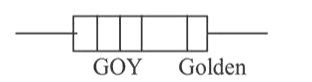# A carbon resistance has following colour code. What is the value of the resistance?Question:

A carbon resistance has following colour code. What is the value of the resistance?1. (1) $530 \mathrm{k} \Omega \pm 5 \%$

2. (2) $5.3 \mathrm{k} \Omega \pm 5 \%$

3. (3) $6.4 \mathrm{M} \Omega \pm 5 \%$

4. (4) $64 \mathrm{M} \Omega \pm 10 \%$

Correct Option: 1

Solution:

(1) Colour code for carbon resistor

Bl, Br, R, O, Y, G, Blue, V, Gr, W

$\begin{array}{llllllllll}0 & 1 & 2 & 3 & 4 & 5 & 6 & 7 & 8 & 9\end{array}$

Resistance, $\mathrm{R}=\mathrm{AB} \times \mathrm{C} \pm \mathrm{D}$

Bands $\mathrm{A}$ and $\mathrm{B}$ are the first two significant figures of resistance

$\mathrm{B}$ and $\mathrm{C}$ indicates the decimal multiplier or the number of zeros that follow A and B

$\mathrm{B}$ and $\mathrm{D}$ is tolerance: Gold $=\pm 5 \%$,

Silver $=\pm 10 \%$ No colour $=\pm 20 \%$

$R=53 \times 10^{4} \pm 5 \%=530 \mathrm{k} \Omega \pm 5 \%$